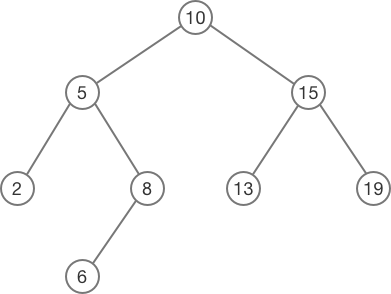#2018 iT 邦幫忙鐵人賽DAY 11
0
Software Development

## 本章重點

• 在 FP 中使用 `Algebraic Data Types` 定義資料結構。
• type 是 `將已有的 Type 組合在一起，然後重新命名` ，而 data 是 `創造新的 Type`
• Type 可以帶有參數，使它可以由不同種 Type 組成，如 `Array a`
• Type 可以是 recursive ， 像是 `Linked list 、 Binary search tree`
• FP 從 `數學與範疇論` 中擷取了不同種的 Class ，它們像是 OOP 的 interface，當 instance 某個 Class ，必須實作某些方法，而他必須符合某些 `法則 (Law)`

## Algebraic Data Types

Maybe Maybe 的機率， Either 恨或愛 中，我們自己創造了新的資料結構，並且讓它有一些我的在 Array 上學的特性。

``````// data Boolean = False | True
``````

``````// data Shape = Circle Number Number Number
``````

### Record

Record 可以賦予每個屬性一點語意，讓我們直接看語法。

``````// data Shape = Circle { x :: Number, y :: Number, radius :: Number }
``````

### Type

``````// data Shape = Circle { x :: Number, y :: Number, radius :: Number }
//            | Rectangle { x1 :: Number, y1 :: Number, x2 :: Number, y2 :: Number }
``````

`{ x :: Number, y :: Number }` 不斷重複，可以使用 Type 降低重複的部份︰

``````// type Point = { x :: Number, y :: Number }
// data Shape = Circle Point Number
//            | Rectangle Point Point
``````

type 是 `將已有的 Type 組合在一起，然後重新命名` ，而 data 是 `創造新的 Type`

``````// type String = Array Char
``````

### 如何實作

``````// type Point = { x :: Number, y :: Number }
// data Shape = Circle Point Number
//            | Rectangle Point Point
class Circle {
constructor(p, r) {
this._p = p
this._r = r
}
}

class Rectangle {
constructor(p0, p1) {
this._p0 = p0
this._p1 = p1
}
create(p0, p1) {
return new Rectangle(p0, p1)
}
}

// showShape :: Shape -> String
const showShape = shape => {
if (shape instanceof Circle) {
// 取出 _vaule0 當中的 x, y
// 與 const [x,...xs] = shape._v1alue0 類似
const { x, y } = shape.p
const r = shape.r

return `Circle (\${x}, \${y}) \${r}`
} else if (shape instanceof Rectangle) {
// 把 x, y 取出後，重新命名（在 : 之後的名稱）
const { x: x1, y: y1 } = shape.p0
const { x: x2, y: y2 } = shape.p1

return `Rectangle (\${x1}, \${y1}) (\${x2}, \${y2})`
}
}

const Shape = {
Circle: curry((p, r) => {
return new Circle(p, r)
}),
Rectangle: curry((p0, p1) => {
return new Rectangle(p0, p1)
})
}

const circle = Shape.Circle({ x: 0, y: 0 }, 10)
const rect = Shape.Rectangle({ x: 0, y: 0 }, { x: 100, y: 100 })
console.log(
showShape(circle), // Circle (0, 0) 10
showShape(rect) // Rectangle (0, 0) (100, 100)
)
``````

• Circle 的 Data Constructor 接受兩個參數， Rectangle 也是。
• `Data Constructor 是可以 Curry 的`
• 在 showShape 的 Type Signature 中，型別是放 `Shape`
• 在實作中，先判斷是 Circle 或 Rectangle ，然後採取各自的實作。

## Type parameters

``````// data Maybe a = Nothing | Just a
//
// Just 1 :: Maybe Int
// Just 8.7 :: Maybe Number
// Just "Monica" :: Maybe String
// Nothing :: Maybe a
``````

``````// 只有 Number 可以 + 1
// divdeMaybe :: Number a => Maybe a -> a -> Maybe a
const incMaybe = maybe => maybe.map(x => x + 1))
``````

## Recursive data structures

``````// data List a = Nil | Cons a (List a)
``````

Nil 代表一個空的節點，而 `Cons` 的 constructor 則有兩個參數︰

• a
這個節點的值。
• List a
連結到下一個 List，如果這是最後一個節點，連接一個 `Nil`

``````// data List a = Nil
//             | Cons a (List a)
class Nil {
constructor() {}
append(a) {
return new Cons(a, this)
}
toString() {
return 'Nil'
}
}

class Cons {
constructor(a, rest) {
this._a = a
this._rest = rest
}
append(a) {
return new Cons(a, this)
}
toString() {
const a = this._a
const rest = this._rest

return `Cons(\${a}, \${rest.toString()})`
}
}

const List = {
Nil: _ => new Nil(),
Cons: curry((a, rest) => new Cons(a, rest))
}

const myList = List.Nil()
.append(613)
.append(69)
.append(42)
console.log(myList.toString())

// Cons(42, Cons(69, Cons(613, Nil)))
```````Binary search tree` 底下共有兩個節點，值較小的放左邊，較大放右邊，最後一個節點則連接兩個 Nil 。``````// data Tree a = Nil
//             | Node a (Tree a) (Tree a)
class Nil {
constructor() {}
insert(a) {
return new Node(a, new Nil(), this)
}
search(_) {
return false
}
toString() {
return 'Nil'
}
}

class Node {
constructor(a, left, right) {
this._a = a
this._left = left
this._right = right
}
insert(b) {
const a = this._a
const left = this._left
const right = this._right

if (b < a) {
return new Node(a, left.insert(b), right)
} else {
return new Node(a, left, right.insert(b))
}
}
search(b) {
const a = this._a
const left = this._left
const right = this._right

if (b == a) {
return true
} else if (b < a) {
return left.search(b)
}
else{
return right.search(b)
}
}
toString() {
const a = this._a
const left = this._left
const right = this._right

return `Node(\${a}, \${left.toString()}, \${right.toString()})`
}
}

const Tree = {
Nil: _ => new Nil(),
Node: curry((a, left, right) => new Node(a, left, right)),
fromArray: array =>
array.reduce(
(tree, a) => tree.insert(a),
new Nil()
)
}

const myTree = Tree.fromArray([8, 6, 1, 7, 9])

console.log(myTree.toString())
// Node(8, Node(6, Node(1, Nil, Nil), Node(7, Nil, Nil)), Node(9, Nil, Nil))
//     8
//    /\
//   6  9
//  /\
// 1  7

console.log(
myTree.search(8), // true
myTree.search(7), // true
myTree.search(0) // false
)
``````

Binary Search Tree 看起來實用多了，主要的概念都來自我們先前的文章。

### Typeclass## 後記